# cosinor2 vignette

#### 2018-10-15

This vignette is intended to be a short guide to using the cosinor2 package. The vignette will briefly walk the interested user through the functions implemented in the package. The cosinor2 package is envisioned as an extension to the previous cosinor package developed by Michael Sachs, but with additional functionalities.

Cosinor is used to fit sine regression models to data. This vignette presumes that the user has theoretical knowledge of cosinor models. To learn more, users are directed to read the article by Cornélissen (2014).

# Population-mean cosinor

The first function in this vignette will be the population.cosinor.lm function which implements the population-mean cosinor in R. Population-mean cosinor is a statistical procedure that implements sine regression (cosinor) to a greater number of individuals, as opposed to the “standard”, single cosinor, that implements it only on one individual/series. Population-mean cosinor procedure consists of fitting single cosinor models to data of each individual separately and averagning cosinor parameters afterwards. However, statistical tests and confidence interval calculation are different than in the case of single cosinor.

The required arguments of the population.cosinor.lm function are:

• data - A data frame that contains subjects’ responses over time. Each row should represent a subject and each column should represent a timepoint at which the data has been collected.
• time - A numeric vector that contains the times at which the data was collected.
• period - A period that will be used in fitting the model (duration of one cycle of rhythm). Cosinor models do not estimate the best-fitting periods like all other cosinor parameters. Instead, the user is expected to provide it.

The optional arguments are:

• na.action - What to do with the missing data. Default is na.omit.
• alpha - Significance level for calculating confidence intervals of cosinor parameters. Defaults to .05.
• plot - Whether or not you want to display the plot after the analysis. Defaults to TRUE.

The function can be used as follows:

fit.panas.cosinor <- population.cosinor.lm(data = PANAS_november, time = PANAS_time, period = 7)
#>      MESOR Amplitude Acrophase
#> 1 1.435419 0.2662682 -5.544496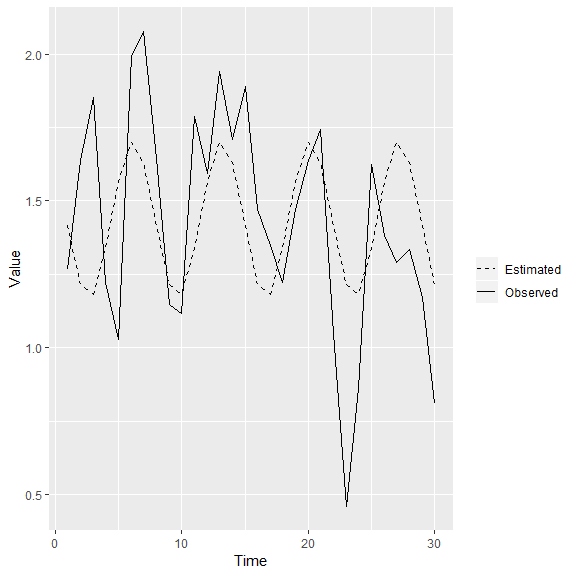The output shows the cosinor parameters and the graph. The coefficients can be accessed by fit.panas.cosinor$coefficients. If the user requires, they can also access more information on the fitted model. For example, the confidence intervals of the parameters would be retrieved by: fit.panas.cosinor$conf.ints
#>                 MESOR  Amplitude Acrophase
#> Upper limit 1.9554141 0.45592967 -4.445865
#> Lower limit 0.9154241 0.07660673 -6.305227

It must be noted that the confidence interval of the acrophase cannot be calculated if the confidence interval of the amplitude includes 0. The user may also access the observed mean of the data, fitted values or residuals by:

fit.panas.cosinor$emp.mean #> X01 X02 X03 X04 X05 X06 X07 #> 1.2656250 1.6388889 1.8541667 1.2222222 1.0294118 1.9916667 2.0789474 #> X08 X09 X10 X11 X12 X13 X14 #> 1.6458333 1.1458333 1.1180556 1.7890625 1.5921053 1.9407895 1.7105263 #> X15 X16 X17 X18 X19 X20 X21 #> 1.8888889 1.4705882 1.3472222 1.2205882 1.4722222 1.6388889 1.7434211 #> X22 X23 X24 X25 X26 X27 X28 #> 1.0486111 0.4583333 0.8618421 1.6250000 1.3815789 1.2894737 1.3333333 #> X29 X30 #> 1.1710526 0.8088235 fit.panas.cosinor$fitted.values
#>   1.417993 1.216824 1.180261 1.335837 1.566401 1.698332 1.632285
#>   1.417993 1.216824 1.180261 1.335837 1.566401 1.698332 1.632285
#>  1.417993 1.216824 1.180261 1.335837 1.566401 1.698332 1.632285
#>  1.417993 1.216824 1.180261 1.335837 1.566401 1.698332 1.632285
#>  1.417993 1.216824

fit.panas.cosinor$residuals #> X01 X02 X03 X04 X05 X06 #> -0.15236838 0.42206511 0.67390576 -0.11361515 -0.53698915 0.29333418 #> X07 X08 X09 X10 X11 X12 #> 0.44666245 0.22783995 -0.07099044 -0.06220535 0.45322512 0.02570434 #> X13 X14 X15 X16 X17 X18 #> 0.24245699 0.07824139 0.47089550 0.25376446 0.16696131 -0.11524914 #> X19 X20 X21 X22 X23 X24 #> -0.09417870 -0.05944360 0.11113613 -0.36938227 -0.75849044 -0.31841880 #> X25 X26 X27 X28 X29 X30 #> 0.28916262 -0.18482197 -0.40885880 -0.29895159 -0.24694075 -0.40800024 Furthermore, all the single cosinors will also be kept after analysis. They are kept in the single.cos list. The user could access the single cosinor analysis object of the sixth subject by: fit.panas.cosinor$single.cos[]
#> Call:
#> .f(formula = .x[[i]], period = ..2, data = ..1, na.action = ..3)
#>
#>  Raw Coefficients:
#> (Intercept)         rrr         sss
#>   1.3414448  -0.3388631  -0.5666818
#>
#>  Transformed Coefficients:
#> (Intercept)         amp         acr
#>    1.341445    0.660270    1.031865

Single cosinor parameters of all subjects can be accessed as follows:

fit.panas.cosinor$pop.mat #> X1 X2 X3 X4 X5 X6 #> 1 1.1576888 2.67929992 1.1138680 0.56495708 2.57065706 1.3414448 #> 2 -0.4525094 0.33149725 0.6800847 1.03064083 0.02810958 -0.3388631 #> 3 -0.6592897 0.05205926 -0.4491993 0.04172762 0.35670584 -0.5666818 #> X7 X8 X9 X10 X11 X12 #> 1 2.56450532 1.18247236 0.58683524 2.4710208 3.08664809 1.5847422 #> 2 -0.39824438 0.19058804 0.96460443 0.6684201 -0.48717024 0.5829757 #> 3 -0.02026741 -0.02879778 -0.06650511 0.4134668 -0.08928715 -0.7425029 #> X13 X14 X15 X16 X17 X18 #> 1 1.20670560 2.212731526 -1.4203358 0.2065499 2.1034854 0.7212902 #> 2 0.19738833 0.007663496 0.9512714 0.3127176 0.2890218 -0.8202136 #> 3 0.04211185 0.181761539 0.2558844 -0.8089338 -0.1966401 -0.6792010 #> X19 #> 1 1.338396749 #> 2 0.002467911 #> 3 -0.442799405 # Acrophase correction If a single cosinor model was fitted previously, it is desirable to use the correct.acrophase function. This need arises because arcus tangent is used to calculate the acrophase (see the aforementioned article by Cornélissen for details and formulae). However, there can be more angles with the same value of arcus tangent and not all of them are correct. This function can, therefore, be used to place the acrophase in the correct quadrant. For example, if a single cosinor model is fit to the air temperature data: fit.temp.cosinor <- cosinor.lm(Temperature ~ time(Time), period = 24, data = temperature_zg) … the value of the acrophase can be retrieved: fit.temp.cosinor$coefficients
#> (Intercept)         amp         acr
#>   23.083333    6.821466    0.985428

… however, it might not be the appropriate value of the acrophase. Therefore, the correct.acrophase function can be used to put the value in the appropriate quadrant:

correct.acrophase(fit.temp.cosinor)
#>  -4.127021

The output now shows the correct value of the acrophase.

# Rhythm detection test

After fitting the model, the fit of the model should be assessed. This can be done by using the rhythm detection test:

cosinor.detect(fit.panas.cosinor)
#>             F df1 df2          p
#> [1,] 4.732237   2  17 0.02323689

The output shows the F ratio, degrees of freedom and the p-value. If we accept the 5% significance criterion, the tested model would fit to the data.

Rhythm detection test can also be used on single cosinor models, thus extending the cosinor package:

cosinor.detect(fit.temp.cosinor)
#>             F df1 df2            p
#> [1,] 681.1604   2  45 2.282638e-34

This model also fits the data good.

# Percent Rhythm

After the fit of the model is assessed, it can be useful to chech the relative power of the rhythm by checking the proportion of the variance explained by the rhythm (Percent Rhythm). This can be done using the cosinor.PR function:

cosinor.PR(fit.panas.cosinor)
#>           r Percent rhythm     p-value
#> 1 0.4637158      0.2150324 0.009851019

The output shows the correlation between observed and estimated data, coefficient of determination (squared correlation) and the p-value showing if the correlation is significant or not. As previously, the function can also be run on the single cosinor models:

cosinor.PR(fit.temp.cosinor)
#>           r Percent rhythm p-value
#> 1 0.9838823      0.9680243       0

# Periodogram

However, in some cosinor analyses, the users might not have a prior idea on the best-fitting period. In this case, the periodogram can be used to find candidates for the best fitting period. To construct a periodogram, a series of cosinor analyses are conducted, each using a different period. The best-fitting period can be thought of as a period that will maximalize the proportion of explained variance. The periodogram is, then, just a plot showing the proportion of explained variance by each period. This approach has been implemented in the cosinor2 package.

The required arguments to this function are:

• data - A data frame that contains subjects’ responses over time. Each row should represent a subject and each column should represent a timepoint at which the data has been collected.
• time - A numeric vector that contains the times at which the data was collected.

The optional arguments are:

• periods - A vector of periods that the user wants to include in construction of the periodogram. Defaults to the same vector as provided in the time argument.
• na.action - What to do with the missing data. Default is na.omit.
• alpha - Significance level for determining if the model with a given period fits well to the data. Defaults to .05.

The function is used as follows:

periodogram(data = PANAS_november, time = PANAS_time)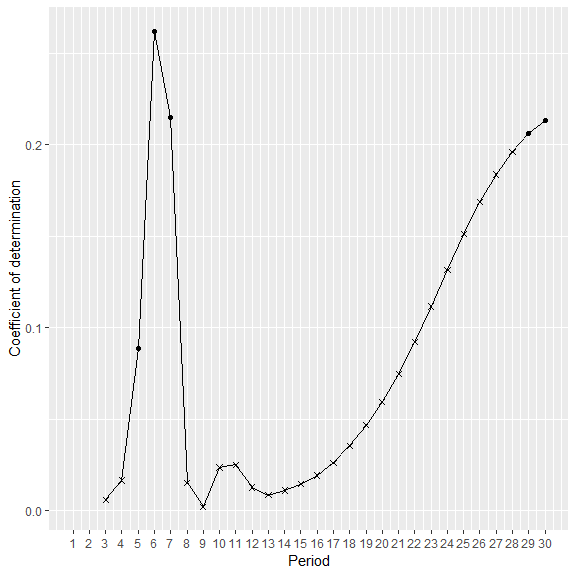The output will inform the user of the best-fitting period. The same information can be seen from the plot. Furthermore, periods with rhythms that fit the data well are represented by dots and periods with rhythms that do not fit the data well are represented by crosses.

In some situations, it can happen that the measurement times are not the times we want test for model fit. For example, the positive affect dataset included in this package contains data that was collected at 10 AM, 12 PM, 2 PM, 4 PM, 6 PM and 8 PM, but the periods that are actually interesting for testing are 1 - 24, since the rhythm is presumed to be circadian. periods argument can be used in such a situation as follows:

periodogram(data = PA_extraverts, time = PA_time, periods = 1:24)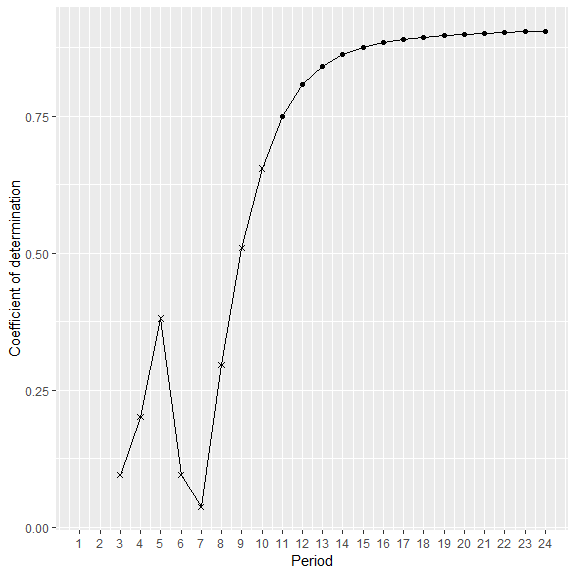Users should note that the first two periods are never tested in the periodogram analysis. This is because the estimated curve doesn’t take on the sinusoidal shape if the number of timepoints is lesser than 3. However, users should not “cut-off” timepoints from the periods argument, as the first two periods will automatically get “cut-off” by the code. In other words, the correct call to argument in the previous example is indeed 1:24 and not 3:24.

Users should also note that, if the midnight is included in their time and/or periods vector, it should be coded as 24 and not as 0.

Like previous functions, it can also be used on the data intended for single cosinor analysis.

# Comparison of cosinor parameters of two populations

Certain users may also wish to compare if the population cosinor parameters of two populations are equal. In order to assess this, models firstly need to be fit to the data:

fit.pa_ext.cosinor <- population.cosinor.lm(data = PA_extraverts, time = PA_time, period = 24)
#>      MESOR Amplitude Acrophase
#> 1 3.053484  0.290861 -4.144953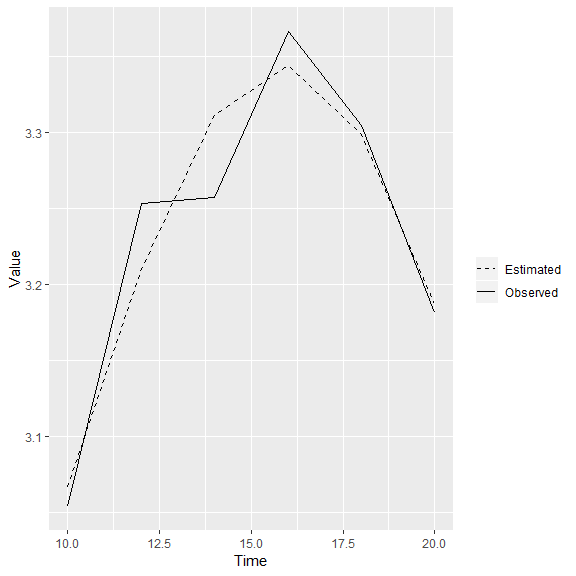fit.pa_int.cosinor <- population.cosinor.lm(data = PA_introverts, time = PA_time, period = 24)
#>      MESOR Amplitude Acrophase
#> 1 2.917414 0.2190473 -5.058308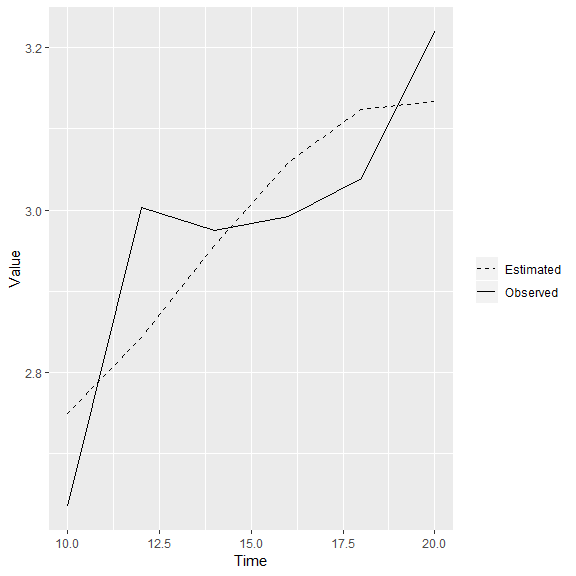Equality of parameters can be compared as follows:

cosinor.poptests(fit.pa_ext.cosinor, fit.pa_int.cosinor)
#>                    F df1 df2         p 1st population 2nd population
#> MESOR     0.32644795   1  51 0.5702677       3.053484      2.9174142
#> Amplitude 0.01669058   1  51 0.8977139       0.290861      0.2190473
#> Acrophase 2.25074729   1  51 0.1397157      -4.144953     -5.0583083

The output shows the F ratio, degrees of freedom and the p-value for MESOR, amplitude and acrophase. For convenience, it also shows the values of the parameters in the two populations.

Users should note that the acrophases of the two populations cannot be compared if their amplitudes are significantly different.

# Serial sections

Lastly, serial sections are a method applied to analyze non-stationary data. Stationary cosinor models presume that the values of cosinor parameters do not change over time. However, in some cases it is evident that those changes are happening. A good example of this is global warming, where the MESOR of the temperature is increasing over time.

To analyze such changes, a cosinor model can be fitted just on a section of the data and calculate cosinor parameters in that section. After that, cosinor model can be fitted on another section of the data. If this process is repeated multiple times, changes in cosinor parameters can be recorded as they change over sections. For easy interpretation, they can be plotted.

There are two key elements to defining serial section analysis. The first one is the interval. The interval is the length of the section of data that will be analyzed while successively fitting the cosinor model to data. The second one is the increment. The increment regulates the number of timepoints by which the interval will be displaced during the analysis.

For example, if the interval is 5 and increment is 1, the cosinor model would first be fit to the data collected at 1st through 5th measurement times. After that, it would be fit to the data collected at 2nd through 6th measurement times etc. Note that in this case, the sections are overlapping - both the first and the second interval contain data collected at 2nd through 5th measurement points.

However, the sections can also be non-overlapping. This is the case when the interval and increment are equal. For example, if both the interval and the increment are 5, the first cosinor model will be fit to the data collected at 1st through 5th measurement points. But, the next interval will be fitted to the 6th through 10th measurement points. In this case, the sections do not overlap. Obviously, the increment cannot be higher than the interval as this results in data loss.

The required arguments to this function are:

• data - A data frame that contains subjects’ responses over time. Each row should represent a subject and each column should represent a timepoint at which the data has been collected.
• time - A numeric vector that contains the times at which the data was collected.
• period - Duration of one cycle of rhythm.
• interval - Length of the interval.
• increment - Length of the increment.

The optional arguments are:

• na.action - What to do with the missing data. Default is na.omit.
• alpha - Significance level for calculating confidence intervals. Defaults to .05.

The function is used as follows:

fit.panas.ssections <- ssections(data = PANAS_november, time = PANAS_time, period = 7, interval = 7, increment = 1)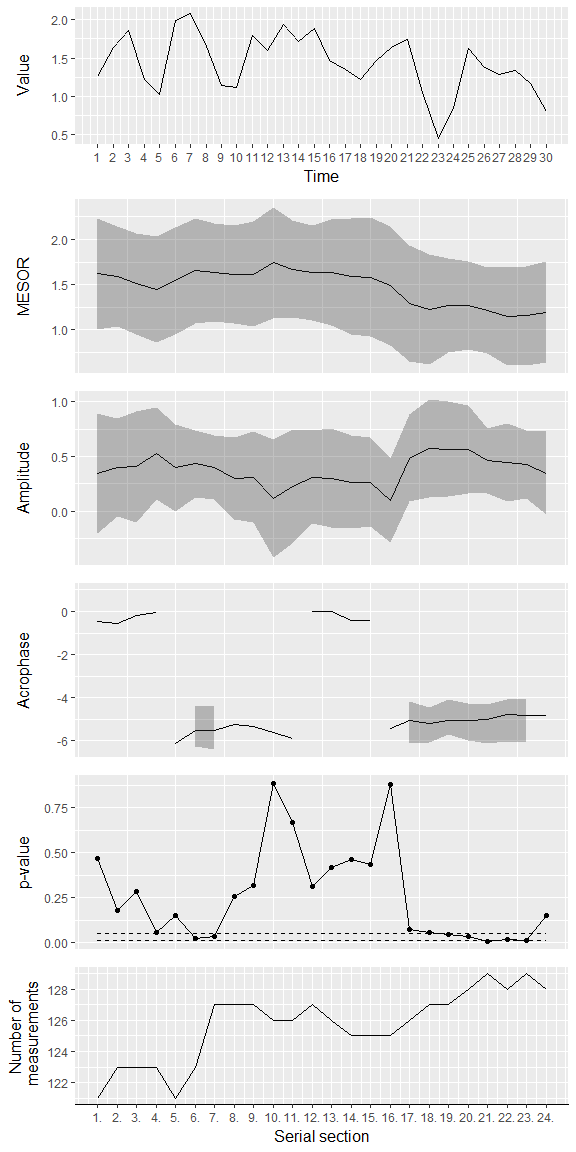The function will generate the plot that is used for interpretation of the serial section analysis (vignette limits the size of the plots, so it is preferrable to look at the above plot after running the function in R). The plot is actually a collection of plots stacked on top of each other. The first plot is the chronogram, showing the observed mean over time.

The following three plots show how MESOR, amplitude and acrophase change over sections. Their confidence intervals are also shown. Users should note that, as mentioned previously, confidence intervals of the acrophase cannot always be calculated. Therefore, the plot of the acrophase may not show confidence intervals in all sections. Furthermore, users should remember that the acrophase is a circular variable. This means that the change from 2°to 358°is not a change of 356°, but a change of 4°.

The fourth plot shows the p-value from the rhythm detection test in each section. Two dashed lines are also shown on the plot, representing the usual .05 and .01 significance levels. The last plot simply shows the number of measurements taken in each section.

Other useful information may also be accessed after analysis. Users may access the empirical mean by calling:

fit.panas.ssections$emp.mean #> emp.mean #> X01 1.2656250 #> X02 1.6388889 #> X03 1.8541667 #> X04 1.2222222 #> X05 1.0294118 #> X06 1.9916667 #> X07 2.0789474 #> X08 1.6458333 #> X09 1.1458333 #> X10 1.1180556 #> X11 1.7890625 #> X12 1.5921053 #> X13 1.9407895 #> X14 1.7105263 #> X15 1.8888889 #> X16 1.4705882 #> X17 1.3472222 #> X18 1.2205882 #> X19 1.4722222 #> X20 1.6388889 #> X21 1.7434211 #> X22 1.0486111 #> X23 0.4583333 #> X24 0.8618421 #> X25 1.6250000 #> X26 1.3815789 #> X27 1.2894737 #> X28 1.3333333 #> X29 1.1710526 #> X30 0.8088235 Coefficients in each section and the p-values of the rhythm detection test can be accessed by: fit.panas.ssections$coefficients
#>       MESOR  Amplitude   Acrophase
#> 1  1.620451 0.34027807 -0.45574371
#> 2  1.587965 0.39820167 -0.57971454
#> 3  1.507859 0.40528865 -0.18091110
#> 4  1.448342 0.52850546 -0.03536664
#> 5  1.541431 0.39483605 -6.11781577
#> 6  1.652325 0.43094036 -5.52384166
#> 7  1.632457 0.39935541 -5.53056159
#> 8  1.611006 0.29722738 -5.27086610
#> 9  1.615804 0.31054815 -5.34423880
#> 10 1.742253 0.11759518 -5.64158574
#> 11 1.668699 0.22430019 -5.92338460
#> 12 1.628744 0.30970362 -0.01173145
#> 13 1.633108 0.29987649 -0.01403355
#> 14 1.587389 0.26543534 -0.41643232
#> 15 1.582527 0.26370170 -0.39838372
#> 16 1.484406 0.09529317 -5.43082884
#> 17 1.291141 0.48390351 -5.06782476
#> 18 1.224621 0.57549624 -5.22207309
#> 19 1.269410 0.56753655 -5.05399665
#> 20 1.266885 0.56481764 -5.07275296
#> 21 1.211507 0.45834920 -5.02932362
#> 22 1.146627 0.44466101 -4.77786967
#> 23 1.155386 0.42524955 -4.85290777
#> 24 1.194246 0.34721447 -4.81778602

fit.panas.ssections$p-values #> pvalues #> 1 0.465272532 #> 2 0.178018244 #> 3 0.284503895 #> 4 0.056264660 #> 5 0.149077742 #> 6 0.020773333 #> 7 0.033455600 #> 8 0.257639457 #> 9 0.316080257 #> 10 0.885504201 #> 11 0.669773613 #> 12 0.310933788 #> 13 0.420217099 #> 14 0.460142828 #> 15 0.431851687 #> 16 0.878070379 #> 17 0.072023022 #> 18 0.054745111 #> 19 0.045713937 #> 20 0.032509830 #> 21 0.008675886 #> 22 0.019995774 #> 23 0.013565124 #> 24 0.153756435 Also, cosinor objects of models calculated in each section are stored in the cosinors object. For example, the cosinor model calcualted on the third section can be accessed by: fit.panas.ssections$cosinors[]
#> $single.cos #>$single.cos[]
#> Call:
#> .f(formula = .x[[i]], period = ..2, data = ..1, na.action = ..3)
#>
#>  Raw Coefficients:
#> (Intercept)         rrr         sss
#>   1.8545741  -0.7490001  -0.2806725
#>
#>  Transformed Coefficients:
#> (Intercept)         amp         acr
#>   1.8545741   0.7998614   0.3585335
#>
#> $single.cos[] #> Call: #> .f(formula = .x[[i]], period = ..2, data = ..1, na.action = ..3) #> #> Raw Coefficients: #> (Intercept) rrr sss #> 2.8392857 1.2775382 0.3991111 #> #> Transformed Coefficients: #> (Intercept) amp acr #> 2.8392857 1.3384295 0.3027996 #> #>$single.cos[]
#> Call:
#> .f(formula = .x[[i]], period = ..2, data = ..1, na.action = ..3)
#>
#>  Raw Coefficients:
#> (Intercept)         rrr         sss
#>   0.8571429   1.8287628   0.4804843
#>
#>  Transformed Coefficients:
#> (Intercept)         amp         acr
#>   0.8571429   1.8908301   0.2569304
#>
#> $single.cos[] #> Call: #> .f(formula = .x[[i]], period = ..2, data = ..1, na.action = ..3) #> #> Raw Coefficients: #> (Intercept) rrr sss #> 0.5813546 2.1302333 -0.7896087 #> #> Transformed Coefficients: #> (Intercept) amp acr #> 0.5813546 2.2718661 -0.3549671 #> #>$single.cos[]
#> Call:
#> .f(formula = .x[[i]], period = ..2, data = ..1, na.action = ..3)
#>
#>  Raw Coefficients:
#> (Intercept)         rrr         sss
#>   2.5390739  -0.0284006   1.1219178
#>
#>  Transformed Coefficients:
#> (Intercept)         amp         acr
#>    2.539074    1.122277   -1.545487
#>
#> $single.cos[] #> Call: #> .f(formula = .x[[i]], period = ..2, data = ..1, na.action = ..3) #> #> Raw Coefficients: #> (Intercept) rrr sss #> 1.401745 -0.107051 1.143905 #> #> Transformed Coefficients: #> (Intercept) amp acr #> 1.401745 1.148903 -1.477484 #> #>$single.cos[]
#> Call:
#> .f(formula = .x[[i]], period = ..2, data = ..1, na.action = ..3)
#>
#>  Raw Coefficients:
#> (Intercept)         rrr         sss
#>   3.3972509   0.2516731  -0.7694621
#>
#>  Transformed Coefficients:
#> (Intercept)         amp         acr
#>   3.3972509   0.8095748  -1.2546874
#>
#> $single.cos[] #> Call: #> .f(formula = .x[[i]], period = ..2, data = ..1, na.action = ..3) #> #> Raw Coefficients: #> (Intercept) rrr sss #> 1.125000000 -0.004492044 1.386773353 #> #> Transformed Coefficients: #> (Intercept) amp acr #> 1.125000 1.386781 -1.567557 #> #>$single.cos[]
#> Call:
#> .f(formula = .x[[i]], period = ..2, data = ..1, na.action = ..3)
#>
#>  Raw Coefficients:
#> (Intercept)         rrr         sss
#>   0.6071429   1.2137260   0.1424947
#>
#>  Transformed Coefficients:
#> (Intercept)         amp         acr
#>   0.6071429   1.2220620   0.1168677
#>
#> $single.cos[] #> Call: #> .f(formula = .x[[i]], period = ..2, data = ..1, na.action = ..3) #> #> Raw Coefficients: #> (Intercept) rrr sss #> 2.42857143 -0.04577355 0.78763242 #> #> Transformed Coefficients: #> (Intercept) amp acr #> 2.4285714 0.7889614 -1.5127462 #> #>$single.cos[]
#> Call:
#> .f(formula = .x[[i]], period = ..2, data = ..1, na.action = ..3)
#>
#>  Raw Coefficients:
#> (Intercept)         rrr         sss
#>  3.30357143 -0.05819752 -0.42062486
#>
#>  Transformed Coefficients:
#> (Intercept)         amp         acr
#>   3.3035714   0.4246319   1.4333095
#>
#> $single.cos[] #> Call: #> .f(formula = .x[[i]], period = ..2, data = ..1, na.action = ..3) #> #> Raw Coefficients: #> (Intercept) rrr sss #> 1.5714286 0.9870828 -0.8019290 #> #> Transformed Coefficients: #> (Intercept) amp acr #> 1.5714286 1.2717793 -0.6822703 #> #>$single.cos[]
#> Call:
#> .f(formula = .x[[i]], period = ..2, data = ..1, na.action = ..3)
#>
#>  Raw Coefficients:
#> (Intercept)         rrr         sss
#>   1.4482183  -0.6536322   0.5156570
#>
#>  Transformed Coefficients:
#> (Intercept)         amp         acr
#>   1.4482183   0.8325486  -0.6679422
#>
#> $single.cos[] #> Call: #> .f(formula = .x[[i]], period = ..2, data = ..1, na.action = ..3) #> #> Raw Coefficients: #> (Intercept) rrr sss #> 1.6708234 0.0572453 0.1736328 #> #> Transformed Coefficients: #> (Intercept) amp acr #> 1.670823 0.182826 1.252327 #> #>$single.cos[]
#> Call:
#> .f(formula = .x[[i]], period = ..2, data = ..1, na.action = ..3)
#>
#>  Raw Coefficients:
#> (Intercept)         rrr         sss
#>   -1.154425    1.454080    1.106835
#>
#>  Transformed Coefficients:
#> (Intercept)         amp         acr
#>  -1.1544253   1.8274109   0.6506258
#>
#> $single.cos[] #> Call: #> .f(formula = .x[[i]], period = ..2, data = ..1, na.action = ..3) #> #> Raw Coefficients: #> (Intercept) rrr sss #> 0.5714286 0.6563762 -0.7598971 #> #> Transformed Coefficients: #> (Intercept) amp acr #> 0.5714286 1.0041281 -0.8583622 #> #>$single.cos[]
#> Call:
#> .f(formula = .x[[i]], period = ..2, data = ..1, na.action = ..3)
#>
#>  Raw Coefficients:
#> (Intercept)         rrr         sss
#>    2.660714    1.273229   -1.038261
#>
#>  Transformed Coefficients:
#> (Intercept)         amp         acr
#>    2.660714    1.642893   -0.684094
#>
#> $single.cos[] #> Call: #> .f(formula = .x[[i]], period = ..2, data = ..1, na.action = ..3) #> #> Raw Coefficients: #> (Intercept) rrr sss #> 0.7321429 -2.4721199 -0.6558406 #> #> Transformed Coefficients: #> (Intercept) amp acr #> 0.7321429 2.5576364 0.2593212 #> #>$single.cos[]
#> Call:
#> .f(formula = .x[[i]], period = ..2, data = ..1, na.action = ..3)
#>
#>  Raw Coefficients:
#> (Intercept)         rrr         sss
#>   0.2142857   0.5635347  -0.3566306
#>
#>  Transformed Coefficients:
#> (Intercept)         amp         acr
#>   0.2142857   0.6669008  -0.5642214
#>
#>
#> $pop.mat #> X1 X2 X3 X4 X5 X6 #> 1 1.8545741 2.8392857 0.8571429 0.5813546 2.5390739 1.401745 #> 2 -0.7490001 1.2775382 1.8287628 2.1302333 -0.0284006 -0.107051 #> 3 -0.2806725 0.3991111 0.4804843 -0.7896087 1.1219178 1.143905 #> X7 X8 X9 X10 X11 X12 #> 1 3.3972509 1.125000000 0.6071429 2.42857143 3.30357143 1.5714286 #> 2 0.2516731 -0.004492044 1.2137260 -0.04577355 -0.05819752 0.9870828 #> 3 -0.7694621 1.386773353 0.1424947 0.78763242 -0.42062486 -0.8019290 #> X13 X14 X15 X16 X17 X18 #> 1 1.4482183 1.6708234 -1.154425 0.5714286 2.660714 0.7321429 #> 2 -0.6536322 0.0572453 1.454080 0.6563762 1.273229 -2.4721199 #> 3 0.5156570 0.1736328 1.106835 -0.7598971 -1.038261 -0.6558406 #> X19 #> 1 0.2142857 #> 2 0.5635347 #> 3 -0.3566306 #> #>$coefficients
#>      MESOR Amplitude  Acrophase
#> 1 1.507859 0.4052887 -0.1809111
#>
#> $emp.mean #> X03 X04 X05 X06 X07 X08 X09 #> 1.854167 1.222222 1.029412 1.991667 2.078947 1.645833 1.145833 #> #>$fitted.values
#>  1.180306 1.117027 1.348052 1.699416 1.906534 1.813442 1.490240
#>
#> $residuals #> X03 X04 X05 X06 X07 X08 #> 0.6738608 0.1051957 -0.3186407 0.2922505 0.1724135 -0.1676082 #> X09 #> -0.3444063 #> #>$conf.ints
#>                 MESOR  Amplitude Acrophase
#> Upper limit 2.0696275  0.9139306        NA
#> Lower limit 0.9460913 -0.1033533        NA
#>
#> attr(,"class")
#>  "population.cosinor.lm"

This function can also be used with data intended to be analyzed by single cosinor.

Users should note that R performs listwise deletion while calculating regression models and therefore results may differ from other software packages that use pairwise deletion.